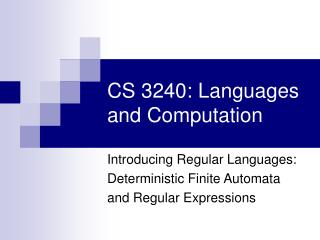# CS 3240: Languages and Computation - PowerPoint PPT PresentationDownload PresentationCS 3240: Languages and Computation

CS 3240: Languages and Computation
Download Presentation## CS 3240: Languages and Computation

- - - - - - - - - - - - - - - - - - - - - - - - - - - E N D - - - - - - - - - - - - - - - - - - - - - - - - - - -
##### Presentation Transcript

1. CS 3240: Languages and Computation Introducing Regular Languages: Deterministic Finite Automata and Regular Expressions

2. Automata • Finite Automata model many design and analysis tasks, e.g. • Lexical analyzer in a compiler • Digital cicuit design • Keywork searching in texts or on the web. • Software for verifying finite state systems, such as communication protocols. • Your ATM, vendig machine, Etc. • 1950-59 McCulloch&Pitts, Rabin&Scott, Moore, Huffman

3. Finite State Machine and Finite Automata

4. Deterministic Finite Automata • A simplest model for computing • Deterministic: Machine is in a state. Upon receipt of a symbol will go to a unique state. • Finite: Have a finite number of states • Automata: (pl. of automaton) Self-operating machine • DFA: finite-state machine without ambiguity

5. DFA and Strings • DFA can recognize strings • String is input • If DFA ends at accept state, string is recognized • A language is called a regular language if some finite automaton recognizes it • Let us look at a few example before giving formal mathematical definition

6. 2 DFA Examples 0 1 1 start state 1 accept state 0 transition Note: The alphabet for this example is {0, 1}. Each state has a transition for every symbol in the alphabet Accept all strings that end in 1

7. 1 3 2 a b a b b b a a 4 5 b a DFA Examples Accept strings of 'a's and 'b's that begin and end with same symbol

8. 0 DFA Examples 0 1 Start 2 1 2 0 1 2 0 2 Keep running count of total of symbols read in mod 3. Accept on 0. 1

9. 0 0 1 Odd Even 1 DFA Examples Strings with an odd number of ones.

10. 0,1 1 1 0 0 '001' '0' '00' 1 0 DFA Examples Strings containing the substring 001

11. Formal Definition of DFA • A DFA consists of: • Alphabet • A set of statesQ • A transition functionδ : Q  Q • One start state q0 • One or more accepting states F  Q • Language accepted by a DFA is the set of strings such that DFA ends at an accepting state • Each string is c1c2…cn with ci   • States are qi = δ(qi-1,ci ) for i=1…n • qn is an accepting state

12. Examples • Design a DFA to recognize strings that start out with k zeros followed by k ones. • Impossible • Design a DFA to recognize strings with an equal number of ones and zeros. • Impossible • Design a DFA to recognize strings with an equal number of strings "01" and "10". • Possible!

13. Actually the thirdone is regular! 0 1 1 1 0 0 0 1 1 0 1 0 DFA to recognize strings with an equal number of strings "01" and "10" 0 1

14. DFA More examples

15. DFA More examples A,B are the input into which the marble is dropped. The x-levers cause fall either to left or right, but lever reverses upon a marble passing Accept if marble exits through D

16. Non-Deterministic Finite Automata

17. q2 1  q1 q1 q2 q3 1 empty string transition nondeterministic transition NFA and -NFA • Nondeterministic Finite Automata • Same input may produce multiple paths • Allows transition with an empty string or transition from one state to different states given a character